# PSAT Math : How to factor a trinomial

## Example Questions

### Example Question #1 : How To Factor A Trinomial

Factor the following expression completely: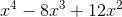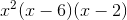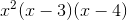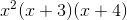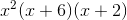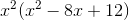Explanation:

We must begin by factoring outfrom each term.Next, we must find two numbers that sum toand multiply to.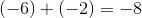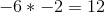### Example Question #2 : How To Factor A Trinomial

Factor the following trinomial: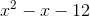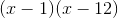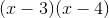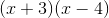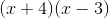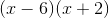Explanation:To trinomial is in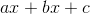form

In order to factor, find two numbers whose procuct is, in this case, and whose sum is, in this caseFactors of: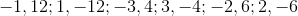Which of these pairs has a sum of?andTherefore the factored form ofis:### Example Question #3 : How To Factor A Trinomial

Factor the trinomial.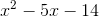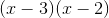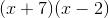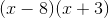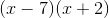Our factors will need to have a product of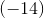, and a sum of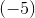, so our factors must be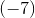and.